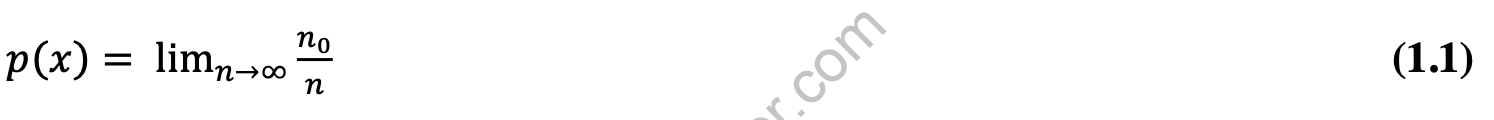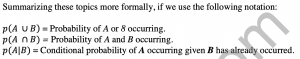# Decisions Under Risk: Probability and ExpectationThere are as many different reasons for playing poker as there are players who play the game. Some play for social reasons, to feel part of a group or “one of the guys,” some play for recreation, just to enjoy themselves. Many play for the enjoyment of competition. Still others – lay to satisfy gambling addictions or to cover up other pain in their lives. One of the difficulties of taking a mathematical approach to these reasons is that it’s difficult to quantify the value of having fun or of a sense of belonging.

In addition to some of the more nebulous and difficult to quantify reasons for playing poker, there may also be additional financial incentives not captured within the game itself. For example, the winner of the championship event of the World Series of Poker is virtually guaranteed to reap a windfall from endorsements, appearances, and so on, over and above the large first prize.

There are other considerations for players at the poker table as well; perhaps losing an additional hand would be a significant psychological blow. While we may criticize this view as irrational, it must still factor into any exhaustive examination of the incentives to play poker. Even if we restrict our inquiry to monetary rewards, we find that preference for money is non-linear. For most people, winning five million dollars is worth much more (or has much more utility) than a 50°/o chance of winning ten million; five million dollars is life-changing money for most, and the marginal value of the additional five million is much smaller.

In a broader sense, all of these issues are included in the utility theory branch of economics. Utility theorists seek to quantify the preferences of individuals and create a framework under which financial and non-financial incentives can be directly compared. In reality, it is utility that we seek to maximize when playing poker (or in fact, when doing any tiring). However, the use of utility theory as a basis for analysis presents a difficulty; each individual has his own utility curves and so general analysis becomes extremely difficult.

In this book, we will therefore refrain from considering utility and instead use money won inside the game as a proxy for utility. In the bankroll theory section in Part IV, we will take an in-depth look at certain meta-game considerations, introduce such concepts as risk of ruin, the Kelly criterion, and certainty equivalent. All of these are measures of risk that have primarily to do with factors outside the game. Except when expressly stated, however, we will take as a premise that players are adequately bankrolled for the games they are playing in, and that their sole purpose is to maximize the money they will win by making the best decisions at every point.

Maximizing total money won in poker requires that a player maximize the expected value of his decisions. However, before we can reasonably introduce this cornerstone concept, we must first spend some time discussing the concepts of probability that underlie it. The following material owes a great debt to Richard Epstein’s text The Theory of Gambling and Statistical Logic (1967), a valuable primer on probability and gambling.

Probability

Most of the decisions in poker take place under conditions where the outcome has not yet been determined. When the dealer deals out the hand at the outset, the players’ cards are unknown, at least until they are observed. Yet we still have some information about the contents of the other players’ hands. The game’s rules constrain the contents of their hands-while a player may hold the jack-ten of hearts, he cannot hold the ace-prince of billiard tables, for example. The composition of the deck of cards is set before starting and gives us information about the hands.

Consider a holdem hand. What is the chance that the hand contains two aces? You may know the answer already, but consider what the answer means. What if we dealt a million hands just like this? How many pairs of aces would there be? What if we dealt ten million? Over time and many trials, the ratio of pairs of aces to total hands dealt will converge on a particular number. We define probability as this number. Probability is the key to decision-making in poker as it provides a mathematical framework by which we can evaluate the likelihood of uncertain events.

If n trials of an experiment (such as dealing out a holdem hand) produce no occurrences of an event x, we define the probability p of x occurring p{x) as follows:

Now it happens to be the case that the likelihood of a single holdem hand being a pair of aces is 1/221.

We could, of course, deterrnine this by dealing out ten billion hands and observing the

ratio of pairs of aces, to total hands dealt. Tins, however, would be a lengthy and difficult process, and we can do better by breaking the problem up into components. First we consider just one card. What is the probability that a single card is an ace? Even this problem can be broken down further – what is the probability that a single card is the ace of spades?

This final question can be answered rather directly. We make the following assumptions:

• There are fifty-two cards in a standard deck.
• Each possible card is equally likely.

Then the probability of any particular card being the one chosen is 1/52. If the chance of the card being the ace of spades is 1/52, what is the chance of the card being any ace? This is equivalent to the chance that the card is the ace of spades OR that it is the ace of hearts OR that it is the ace of diamonds OR that it is the ace of clubs. There are four aces in the deck, each with a 1/52 chance of being the card, and summing these probabilities, we have:

We can sum these probabilities directly because they are mutually exclusive; that is, no card can simultaneously be both the ace of spades and the ace of hearts. Note that the probability 1/13 is exactly equal to the ratio (number of aces in the deck)/(number of cards total). This relationship holds just as well as the summing of the individual probabilities.

Independent Events

Some events, however, are not mutually exclusive. Consider for example, these two events:

1. The card is a heart
2. The card is an ace.

If we try to figure out the probability that a single card is a heart OR that it is an ace, we find there are thirteen hearts in the deck out of fifty-cards, so the chance that the card is a heart is 1/4. The chance that the card is an ace is, as before, 1/13.

However, we cannot simply add these probabilities as before, because it is possible for a card to both be an ace and a heart.
There are two types of relationships between events. The first type are events that have no effect on each other. For example, the closing value of the NASDAQ stock index and the value of the dice on a particular roll at the craps table in a casino in Monaco that evening are basically unrelated events; neither one should impact the other in any way that is not negligible. If the probability of both events occurring equals the product of the individual probabilities, then the events are said to be independent. The probability that both A and B occur is called the joint probability of A and B.

In this case, the joint probability of a card being both a heart and an ace is(1/13)(1/4),or 1/52. This is because the fact that the card is a heart does not affect the chance that it is an ace – all four suits have the same set of cards.

Independent events are not mutually exclusive except when one of the events has probability zero. In this example, the total number of hearts in the deck is thirteen, and the total of aces in the deck is four. However, by adding these together, we are double-counting one single card (the ace of hearts). There are actually thirteen hearts and three other aces, or if you prefer, four aces, and twelve other hearts. It turns out that the general application of this concept is that the probability that at least one of two mutually non-exclusive events A and B will occur is the sum of the probabilities of A and B minus the joint probability of A and B. So the probability of the card being a heart or an ace is equal to the chance of it being a heart ( 1/4 ) plus the chance of it being an ace minus the chance of it being both ( 1/52 ), or 4/13 . This is true for all events, independent or dependent.Previous post Understanding And Avoiding TiltNext post Dependent Events